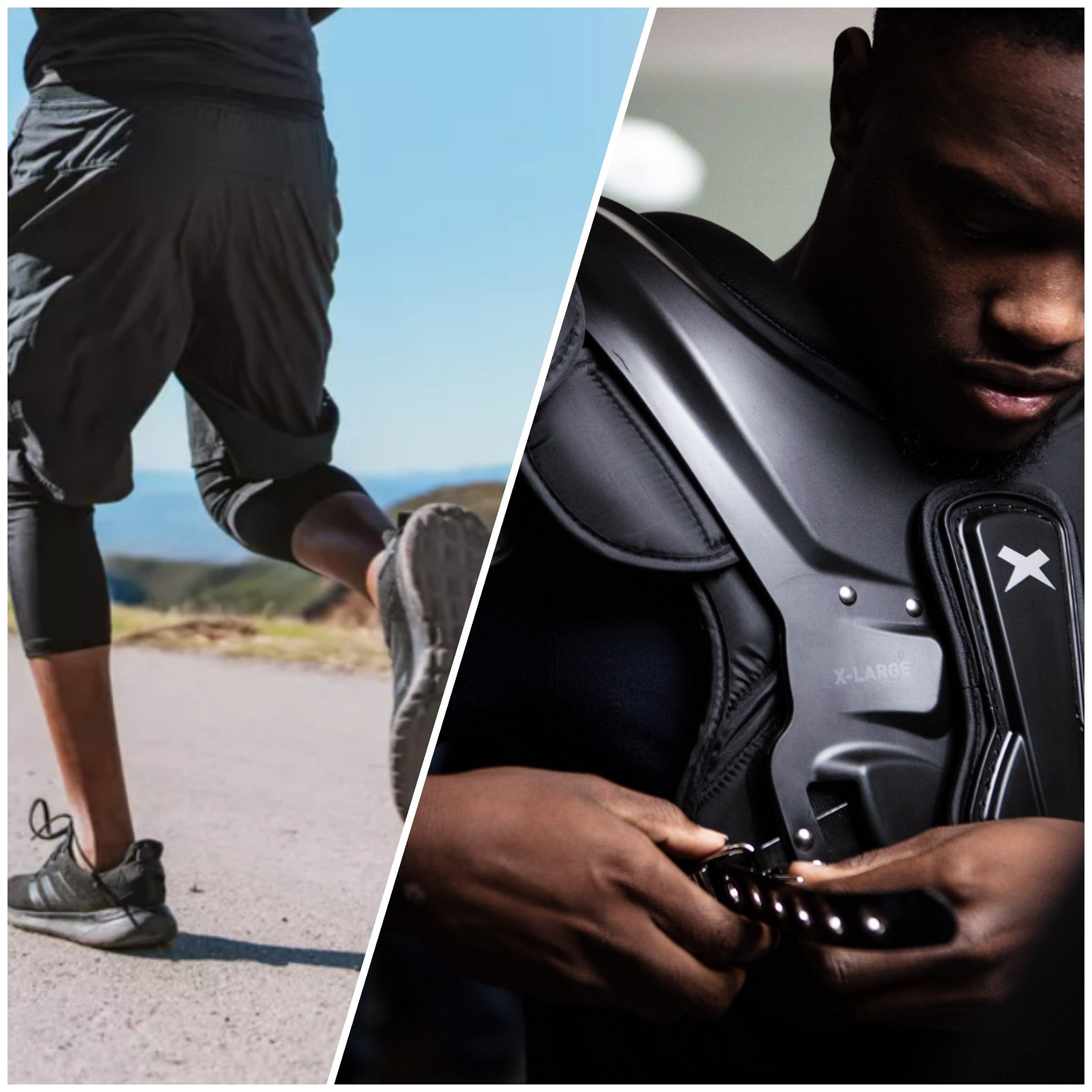# Convolutional Neural Networks — Part 2: Padding and Strided Convolutions

Original article was published by Brighton Nkomo on Artificial Intelligence on Medium# Convolutional Neural Networks — Part 2: Padding and Strided Convolutions

This is the second part of my series of CNN blog posts. Click here for part one (edge detection) of the series.

A pre-requisite here is knowing how to convolve 2 matrices. I have briefly explained how to convolve 2 matrices in my part 1 of this series. Don’t be intimidated by the word convolution and the amount of matrices that you’ll see. I’ve used the asterisk to denote the convolution operator, not matrix multiplication. By the way, matrix convolution is simpler than matrix multiplication.

In plain English, a padding is “the pieces of material used to protect something or give it shape.” The 2 subsections here discuss why it’s necessary to “cover” an input matrix with a border of zeros and the formula for determining the “padding amount.”

# 1.1 Motivation

In earlier post we saw that if you take a 6 by 6 image and convolve it with a 3 by 3 filter, you end up with a 4 by 4 output (with a 4 by 4 matrix), and that’s because the number of possible positions with the 3 by 3 filter, there are only, sort of, 4 by 4 possible positions, for the 3 by 3 filter to fit in your 6 by 6 matrix. And the math turns out that if you have a n by n image and convolve it with an f by f filter, then the dimension of the output will be (n — f+1) by (n — f+1). And so for the 6 by 6 image and the 3 by 3 filter on the left, the output image size would be a (6–3+1) by (6–3+1) which simplifies to a 4 by 4 output.

There are two problems when you convolve a filter to an image:

1. The shrinking output: If every time you apply a convolutional operator, your image shrinks, so you come from 6 by 6 down to 4 by 4 then, you can only do this a few times before your image starts getting really small, maybe it shrinks down to 1 by 1 or something, so maybe, you don’t want your image to shrink every time you detect edges or to set other features on it.

2. Throwing away information from the edges of the image: Looking at the left image, we can see that the green pixel on the upper left can only overlap with one filter when you convolve it, whereas, if you take a pixel in the middle, say the red pixel, then there are a lot of 3 by 3 regions (filters) that overlap with that pixel and so, it’s as if pixels on the corners or on the edges are use much less used to compute the output pixels. Hence, you’re throwing away a lot of the information near the edges of the image.

In order to solve both of these problems, both the shrinking output and throwing away a lot of the information from the edges of the image,

what you can do is before applying the convolutional operation, you can pad the image with an additional one border, with the additional border of one pixel all around the edges as shown on the left image(in this case, sometimes you might need more than one border). So, if you do that, then instead of a 6 by 6 image, you’ve now padded this to 8 by 8 image and if you convolve an 8 by 8 image with a 3 by 3 image you now get a 6 by 6 image output. So you managed to preserve the original input size of 6 by 6 (For those familiar with deep learning layer concept: you see that this is especially useful because when you build really deep neural networks, you don’t want the image to shrink on every step because if you have, maybe a 100 layer of deep net, then it’ll shrinks a bit on every layer, then after a hundred layers you end up with a very small image).

By convention when you pad, you padded with zeros and p is the padding amount (in this case, p = 1, because we’re padding all around with an extra boarder of one pixels), then the output becomes (n+2p — f +1) by (n + 2p — f +1). So, in this case we have that the output size when p=1 is (6+2— 3+1) by (6 + 2 — 3+1) which simplifies to just a 6 by 6 image. So you end up with a 6 by 6 image that preserves the size of the original image.

You can also pad the border with two pixels, in that case you add on another border and they can pad it with even more pixels if you choose.